Related Articles
How to create DataFrame from dictionary in Python-Pandas?
• Last Updated : 10 Jul, 2020

Let’s discuss how to create DataFrame from dictionary in Pandas. There are multiple ways to do this task.

Method 1: Create DataFrame from Dictionary using default Constructor of pandas.Dataframe class.

Code:

 `# import pandas library``import` `pandas as pd`` ` `# dictionary with list object in values``details ``=` `{``    ``'Name'` `: [``'Ankit'``, ``'Aishwarya'``, ``'Shaurya'``, ``'Shivangi'``],``    ``'Age'` `: [``23``, ``21``, ``22``, ``21``],``    ``'University'` `: [``'BHU'``, ``'JNU'``, ``'DU'``, ``'BHU'``],``}`` ` `# creating a Dataframe object ``df ``=` `pd.DataFrame(details)`` ` `df`

Output: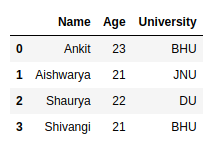Method 2: Create DataFrame from Dictionary with user-defined indexes.

Code:

 `# import pandas library``import` `pandas as pd`` ` `# dictionary with list object in values``details ``=` `{``    ``'Name'` `: [``'Ankit'``, ``'Aishwarya'``, ``'Shaurya'``, ``'Shivangi'``],``    ``'Age'` `: [``23``, ``21``, ``22``, ``21``],``    ``'University'` `: [``'BHU'``, ``'JNU'``, ``'DU'``, ``'BHU'``],``}`` ` `# creating a Dataframe object from dictionary ``# with custom indexing``df ``=` `pd.DataFrame(details, index ``=` `[``'a'``, ``'b'``, ``'c'``, ``'d'``])`` ` `df`

Output: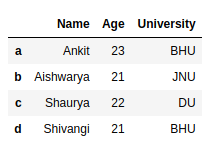Method 3: Create DataFrame from simple dictionary i.e dictionary with key and simple value like integer or string value.

Code:

 `# import pandas library``import` `pandas as pd`` ` `# dictionary``details ``=` `{``    ``'Ankit'` `: ``22``,``    ``'Golu'` `: ``21``,``    ``'hacker'` `: ``23``    ``}`` ` `# creating a Dataframe object from a list ``# of tuples of key, value pair``df ``=` `pd.DataFrame(``list``(details.items()))`` ` `df`

Output: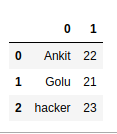Method 4: Create DataFrame from Dictionary with required columns only.

Code:

 `# import pandas library``import` `pandas as pd`` ` `# dictionary with list object in values``details ``=` `{``    ``'Name'` `: [``'Ankit'``, ``'Aishwarya'``, ``'Shaurya'``, ``'Shivangi'``],``    ``'Age'` `: [``23``, ``21``, ``22``, ``21``],``    ``'University'` `: [``'BHU'``, ``'JNU'``, ``'DU'``, ``'BHU'``],``}`` ` `# creating a Dataframe object with skipping ``# one column i.e skipping age column.``df ``=` `pd.DataFrame(details, columns ``=` `[``'Name'``, ``'University'``])`` ` `df`

Output: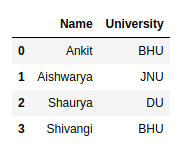Method 5: Create DataFrame from Dictionary with different Orientation i.e. Dictionary key is act as indexes in Dataframe.

Code:

 `# import pandas library``import` `pandas as pd`` ` `# dictionary with list object in values``details ``=` `{``    ``'Name'` `: [``'Ankit'``, ``'Aishwarya'``, ``'Shaurya'``, ``'Shivangi'``],``    ``'Age'` `: [``23``, ``21``, ``22``, ``21``],``    ``'University'` `: [``'BHU'``, ``'JNU'``, ``'DU'``, ``'BHU'``],``}`` ` `# creating a Dataframe object in which dictionary``# key is act as index value and column value is``# 0, 1, 2...``df ``=` `pd.DataFrame.from_dict(details, orient ``=` `'index'``)`` ` `df`

Output: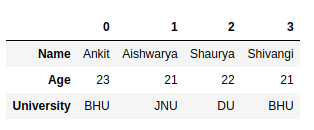Method 6: Create DataFrame from nested Dictionary.

Code:

 `# import pandas library``import` `pandas as pd`` ` `# dictionary with dictionary object``# in values i.e. nested dictionary``details ``=` `{ ``    ``0` `: {``        ``'Name'` `: ``'Ankit'``,``        ``'Age'` `: ``22``,``        ``'University'` `: ``'BHU'``        ``},``    ``1` `: {``        ``'Name'` `: ``'Aishwarya'``,``        ``'Age'` `: ``21``,``        ``'University'` `: ``'JNU'``        ``},``    ``2` `: {``        ``'Name'` `: ``'Shaurya'``,``        ``'Age'` `: ``23``,``        ``'University'` `: ``'DU'``        ``}``}`` ` `# creating a Dataframe object``# from nested dictionary``# in which inside dictionary``# key is act as index value``# and column value is 0, 1, 2...``df ``=` `pd.DataFrame(details)`` ` `# swap the columns with indexes``df ``=` `df.transpose()`` ` `df`

Output: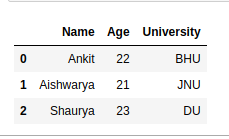Attention geek! Strengthen your foundations with the Python Programming Foundation Course and learn the basics.

To begin with, your interview preparations Enhance your Data Structures concepts with the Python DS Course.

My Personal Notes arrow_drop_up